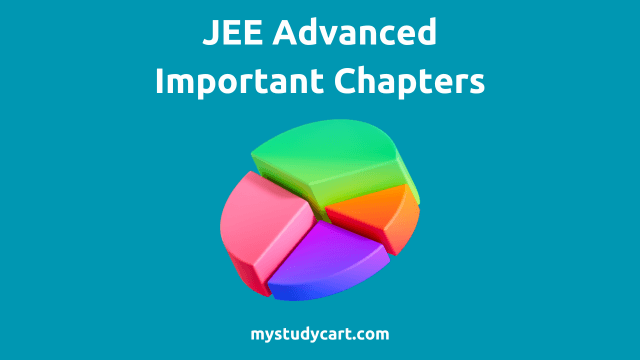# 14 Most Important Chapters for JEE Advanced Physics, Chemistry, Math

By | Last updated on May 3rd, 2023Generally we advise students not to underestimate any chapter for JEE because the exam can surprise everyone, but they keep on asking – What are the most important chapters for JEE Advanced?

Many students are late in starting their preparation, so they want to cover all important chapters required to crack JEE Advanced in the remaining time.

## Most Important Chapters for JEE Advanced Physics, Chemistry, Math

By analyzing previous years papers of JEE Advanced, we can say that there are certain chapters from which the questions are asked in the JEE Advanced every year.

Most Important Chapters for JEE Advanced :

1. Mechanics [Kinematics, Newton’s Laws of Motion, Conservation of Momentum, Work Energy and Power, Gravitation, Rigid Body Dynamics, Simple Harmonic Motion, Fluid Mechanics, Waves].
2. Modern Physics [Photoelectric effect, Bohr H atom, De Broglie wavelength, Nuclear Physics].
3. Electricity and Magnetism [Kirchhoff’s laws, Biot–Savart’s law and Ampere’s law, Electromagnetic induction].
4. Optics [Reflection and refraction, Young’s double-slit experiment].
5. Electrochemistry [Conductivity and conductance, Battery and cell, Faraday’s laws, Nernst equation, Kohlrausch’s law].
6. Thermodynamics [Laws of thermodynamics, Enthalpy, Entropy].
7. Chemical Kinetics [Arrhenius equation, Order of Reaction].
8. Chemical Bonding [VSEPR, Hybridisation, Molecular orbital theory].
9. Coordination Compounds [Nomenclature, Isomerism, Colouration].
10. Matrices and Determinants.
11. Probability and Permutations & Combinations.
12. Co-ordinate Geometry.
13. Definite Integrals.
14. Vectors and 3D Geometry.

## How to Study Important Chapters for JEE Physics?

Tips to study important chapters for JEE Physics :

• Mechanics: Mechanics is the most integral part of JEE Physics. HC Verma and DC Pandey are good books to learn concepts in Mechanics. Go through each and every derivation and understand why each formula holds. Get a feel of how mechanics works applies in real life. Practice questions from your coaching material.
• Modern Physics: Modern Physics has a lot of weightage in JEE and it’s a very scoring part. Don’t skip any topic inside Modern Physics. Follow NCERT and HC Verma/ study modules to read and practice questions in Modern Physics. Keep it in mind not to jump to problem solving without mastering the concept. Solve JEE previous years questions.
• Electricity and Magnetism: Use DC Pandey or Disha book to read and practice questions in electricity and magnetism. Solve previous year JEE questions on electricity and magnetism sincerely.
• Optics: Optics is divided into two parts : Geometrical and Waves optics. Start with NCERT and then refer HC Verma for Optics. Solve problems from your coaching materials, HCV and DCP books. Don’t leave out any topic in optics.

## How to Study Important Chapters for JEE Chemistry?

Tips to study important chapters for JEE Chemistry :

• Electrochemistry: Understand the concept well and read theory of NCERT. Solve Problems in Physical Chemistry for JEE (Main & Advanced) by Narendra Awasthi. Solve as much as questions you can and keep on revising. Remember all the units, formulas and proportionality order which comes in this chapter.
• Thermodynamics: Thermodynamics for Physics and Chemistry is almost the same except for sign convention. Get each concept crystal clear, starting from zeroth law to third law of thermodynamics. When you become confident in theory, move to problems.
• Chemical Kinetics: Chemical kinetics is about formulas and understanding of different cases. This chapter requires practicing a lot of questions. Understand the different types of reactions and how to identify them. Once you know the type of reaction, you should be able to identify the order of reaction and other information.
• Chemical Bonding: Chemical Bonding is an important chapter for both JEE Main and JEE Advanced. Read the entire NCERT chapter and coaching notes. It needs a lot of time and revision every day. Chemical bonding has more theoretical parts and questions get repeated in JEE Main paper. You must solve questions from previous years papers.
• Coordination Compounds: Go through NCERT thoroughly until you understand each and every concept. For practicing problems, refer coaching modules. Make notes for theory with some problems.

## How to Study Important Chapters for JEE Math?

Tips to study important chapters for JEE Math :

• Matrices and Determinants: Matrices and determinants don’t have many formulas or concepts, so you have to practice and practice questions from coaching modules. The more you practice, the more quickly you will solve the question.
• Probability and Permutations & Combinations: Questions regularly come from probability chapter in JEE Main and JEE Advanced. The problems asked in probability involve concepts from permutations and combinations and also binomial theorem. Permutation and combinations requires good logical and thinking skills. Solve problems from the packages provided by coaching and JEE archives.
• Co-ordinate Geometry: Co-ordinate geometry is less about concept and more about how fast you can see the actual trick to solve the problem. Read your coaching notes for theory. Solve questions from coaching material and past year papers. Problems in co-ordinate geometry can be lengthy so avoid calculation mistakes.
• Definite Integrals: Remember all the formulas of definite integral. Questions mainly come from application of formulas, direct indefinite solving then putting the limits, by parts, recursion, area under curves.
• Vectors and 3D Geometry: Both vectors and 3D geometry have good weightage in JEE. Important topics in these units are scalar triple products, vector triple products and some identities. Revise each and every formula from your coaching notes and learn the concept behind them. Do the previous years papers.

Note : It is important that your basics of almost all the chapters are clear and that you can tackle easy or moderate level problems in those chapters, because sometimes easier questions may come from the chapters which you didn’t prepare that well.

Related Post : JEE Main Important Topics Weightage

Master all important JEE topics and chapters by learning from IIT qualified teachers in JEE Online Courses.We’re listening to students.